Publication date: 11/10/2021

# Mixture

The Mixture column property is useful when a column in a data table represents a component of a mixture. The components of a mixture are constrained to sum to a constant. Because of this, they differ from non-mixture factors. The Mixture column property serves two purposes:

It identifies a column as a mixture component.

If you add a column with the Mixture column property as a model effect in the Analyze > Fit Model window, JMP automatically generates a no-intercept model.

It defines the coding for a mixture component.

Coding for mixture components differs from that for non-mixture factors. However, as with non-mixture factors, a benefit of coding for mixture factors is that it helps you interpret parameter estimates. See PseudoComponent Coding.

Figure A.19 shows the Mixture column property panel for the factor m1 in the Vinyl Data.jmp sample data table, found in the Design Experiment folder.

Figure A.19 Mixture Column Property Panel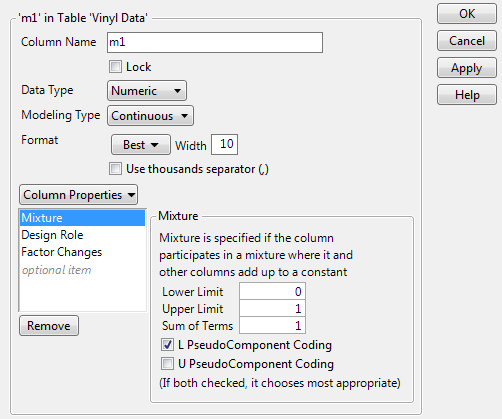In the Mixture column property panel, you can specify the following:

Lower Limit

Specifies the low value used in PseudoComponent Coding. When the Mixture property is applied as part of design construction in a DOE platform, the Lower Limit is set to the minimum setting of the factor. When you apply the Mixture property to a column that does not contain that property, JMP inserts the minimum value as the Lower Limit. You can change this value as needed.

Upper Limit

Specifies the high value used in PseudoComponent Coding. When the Mixture property is applied as part of design construction in a DOE platform, the Upper Limit is set to the maximum setting of the factor. When you apply the Mixture property to a column that does not contain that property, JMP inserts the maximum value as the Upper Limit. You can change this value as needed.

Sum of Terms

Specifies the sum of the mixture components. When you apply the Mixture property to a column that does not contain that property, JMP inserts a default value of 1 for the Sum of Terms.

L PseudoComponent Coding

Transforms data values so that the Lower Limit corresponds to 0.

U PseudoComponent Coding

Transforms data values so that the Upper Limit corresponds to 0.

#### PseudoComponent Coding

A pseudo-component is a linear transformation. Let S denote the sum of the mixture components. Suppose that i columns X1, X2, , Xq have been assigned the Mixture column property. Suppose that the columns and effects constructed from these columns are entered as effects in the Fit Model window.

Define the following: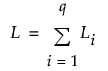, where Li is the Lower Limit for Xi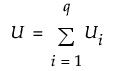, where Ui is the Upper Limit for Xi

Let xi denote a value of the column Xi. The L PseudoComponent at xi is defined as follows: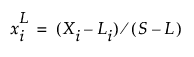The U PseudoComponent at xi is defined as follows: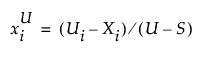If you select both L PseudoComponent Coding and U PseudoComponent Coding, the Fit Model platform uses the L coding if (S L) < (US). Otherwise, the U coding is used.

In Fit Model, mixture factors are transformed using pseudo-components before computing parameter estimates. This helps make parameter estimates more meaningful. In reports dealing with parameter estimates, the mixture main effects are given by the coding transformation. Other reports, such as the profilers, are based on the uncoded values.